Published on

# Big O Notation Made Easy | DSA Series | Part 1 of 3 | Blog Post 1

Author
•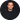Name
Robert Kirby
Title
Lead Full Stack Developer at Y-Squared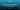## Big O Notation Made Easy

##### Preface

This series will be broken down into three separate blog posts Part 1 Big O Notation, Part 2 Data Structures and Part 3 Algorithms.

The GitHub repo link containing all the code from this post is located at the end of the article!

• 1 Opening Statement
• 2 Brief Introduction to Data structures & Algorithms
• 2.1 What are data structures ?
• 2.2 What are algorithms ?
• 3 What is Big O ?
• 4 O(n) - Linear Time
• 5 O(1) - Constant Time
• 6 Big O Rules
• 6.1 Rule 1: Worst case
• 6.2 Rule 2: Remove constants
• 6.3 Rule 3: Different inputs should have different variables
• 6.4 O(n^2) - Quadratic Time
• 6.5 Rule 4: Drop non-dominant terms
• 7 O(log n) - Logarithmic Time
• 8 O(n log n) - Log Linear Time
• 9 O(2^n) - Exponential Time
• 10 O(n!) - Factorial Time
• 11 Space Complexity - Memory
• 12 What’s Next ?
• 13 Contact, Links & GitHub Repo

## 1 Opening Statement

It is believed that data structures and algorithms are the basics of computer science. It is imperative that we choose the correct combination of data structures and algorithms, doing so we can drastically improve the performance of our programs and applications.

In this post we will do a light introduction to DSA then we will focus on Big O Notation.

## 2.1 Data Structures

A data structure is a way of storing and arranging data on a computer so that it can be accessed and updated efficiently

There are primitive data types and non-primitive data types. During this blog series we will focus on non-primitive data types and in part 2 we will take a deep dive into them but for now here is a quick summary of data types.

Examples of primitive Data Types:Byte, Boolean, Integer, Float, Character

Non-Primitive Data Types:Non primitive data types can be broken into two separate categories:

1 Linear data types - Data elements that are arranged sequentially or linearly.

Examples: String, Array, Queue, Stack, Linked List.

2 Non-Linear data types - Data elements that are not placed sequentially or linearly

Examples: Trees, Graphs.

## 2.2 Algorithms

An algorithm is a list of well designed instructions, used to solve problems or perform tasks.

In part 3 of this series we will dive into algorithms in depth but for now here is a summary of the different types of algorithm in relation to data structures:

Examples: Search Algorithms, Sorting Algorithms, Insertion Algorithms, Update Algorithms, Delete Algorithm

## 3 What is Big O ?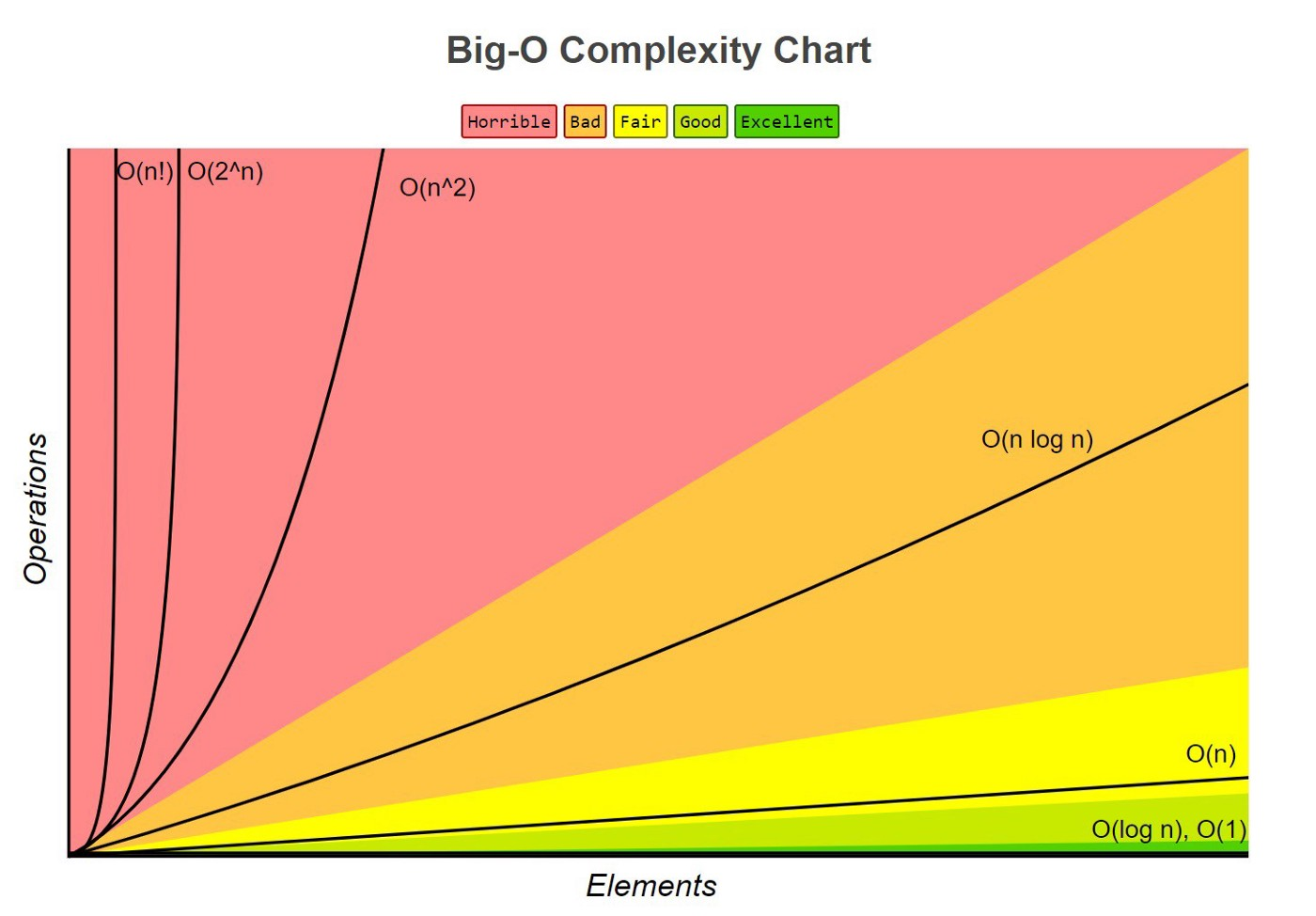Chart source: https://www.bigocheatsheet.com/

Before we jump into DSA in depth it is crucial we understand Big O Notation!

Big O tells us how well a problem is solved and allows us to produce scalable code.

Let’s dive a bit deeper, when we are talking about how well a program is solved we are talking about good code. What constitutes good code? One of the main factors isSPEED!BUT Not all machines are created equally! A program running on your phone, my laptop, a supercomputer at google HQ or on a remote server in the middle of the amazon rainforest will all complete the program tasks at different times. So how do we measure good code with this taken into account ? Big O that’s how!

Big O can be broken down into two categories: time complexity(speed) and space complexity(memory). We’ll get back to space complexity later for now we will just focus on time.

We use Big O to calculate the time complexity or runtime of code not by time itself but rather the number of operations it takes to complete the task.

Now what did we mean by producing scalable code? When our input grows bigger(scaling up) how much does the algorithm slow down? As the amount of elements(inputs) increase, how many more operations do we have to do ? This is called algorithmic efficiency and is explained using Big O by simply comparing n number of inputs with O, the number of operations.

As you can see with the Big O complexity chart at the top of this section, the goal of a programmer is to write as efficient code as possible especially when dealing with scale.

Big O may not matter to you when dealing with a small project with small inputs, but Big O is especially important to large companies dealing with massive scale as algorithmic efficiency is everything and can lead to massive increases in profits with better services and savings on computing power as well as energy needed.

## 4 O(n) - Linear time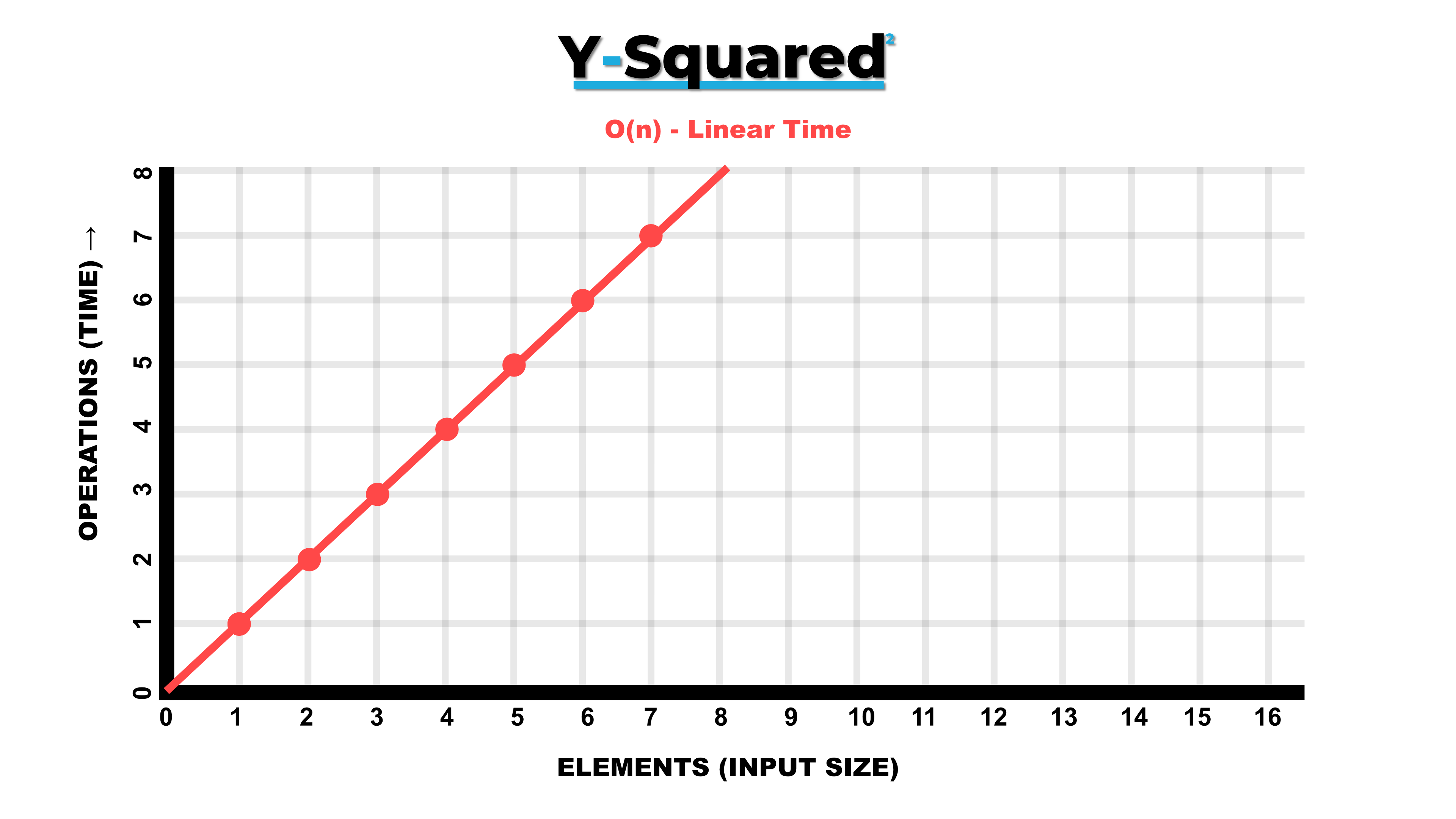O(n) or linear time is one of the most common of the Big O's.

As the number of elements(inputs) increase on the x axis the number of operations(time) on the y axis increase proportionally or linearly!

This means, if there is one input there is one operation, if there are two inputs there are two operations and so on.

Now let’s look at an example! All the examples going forward will be using Javascript but should be easy enough to follow along with once you have a basic understanding of any programming language.

The code below shows a for loop which is checking an array for the car brand Audi. It will loop through the inputs each time increasing the number of operations linearly.

In this case 1 car input = 1 loop operation - 10 car inputs = 10 loop operations, if we go back to the chart above this function would follow the same trajectory.

Example code:

``````const cars = [
"Bugatti", "Ferrari","Lamborghini","Mercedes", "Audi", "Dodge", "Nissan", "Toyota", "Fiat",
"Jaguar",
];

function findAudi(array) {
for (let i = 0; i < array.length; i++) {
if (array[i] === "Audi") {
console.log(`Found Audi at index position \${array.indexOf("Audi")}.`);
}
}
}

findAudi(cars); // 0(n) - Linear Time
``````

O(n) - linear time is rated fair for scalability and will be probably the most common big O you will encounter.

Note: O(n) or Linear Time is usually found with a loop

## 5 O(1) - Constant Time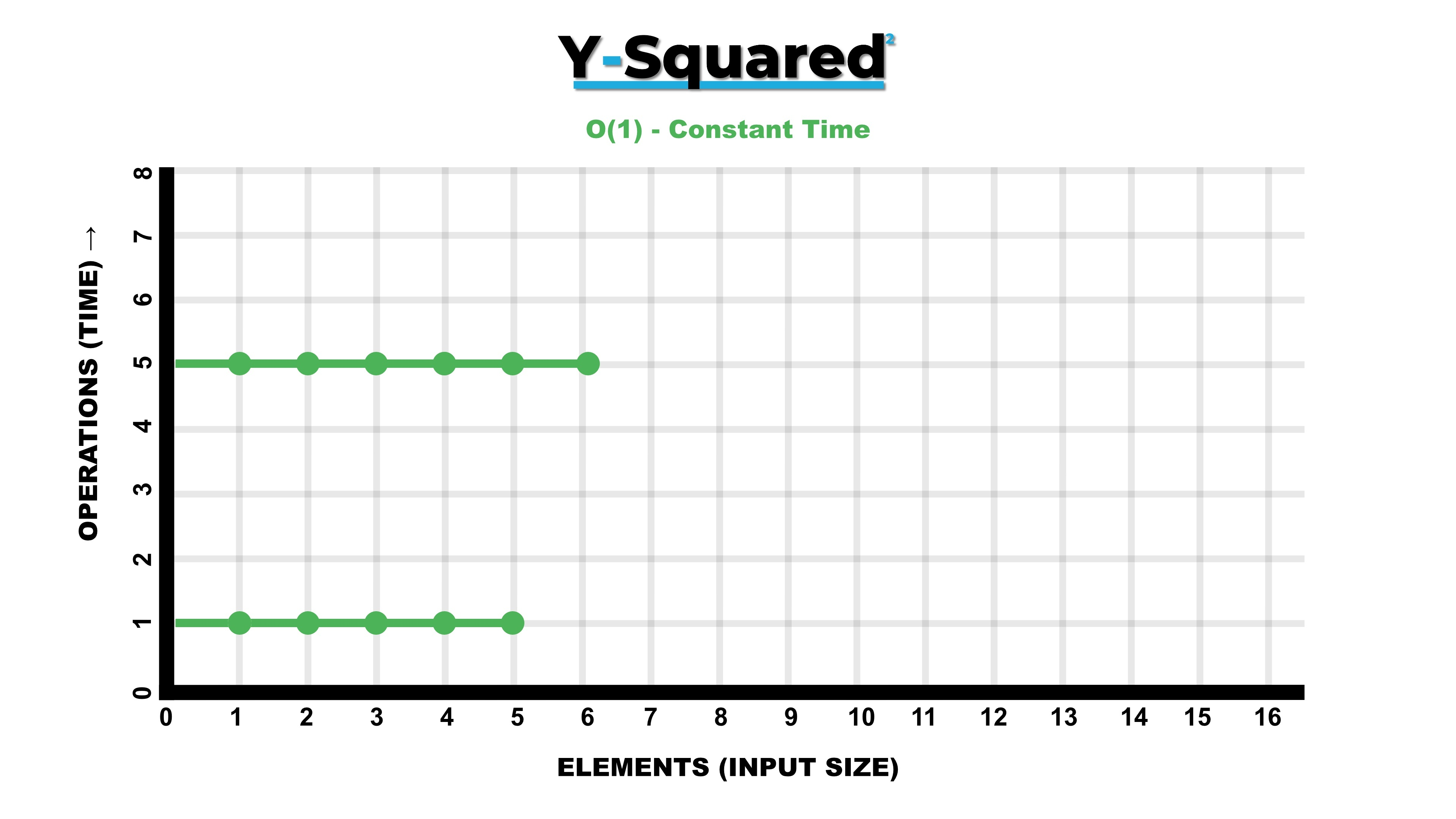O(1) is the other most common of the Big O’s and it means constant time.

As the number of elements(inputs) increase on the x axis the number of operations(time) on the y-axis stays flat or constant.

In the example below we are only performing 1 operation so no matter how many cars are in the array we are still only grabbing the first item in the array meaning there is only 1 operation, this is constant time.

Example code 1:

``````const cars = [
"Bugatti", "Ferrari","Lamborghini","Mercedes", "Audi", "Dodge", "Nissan", "Toyota", "Fiat",  "Jaguar",
];

function findFirst(array) {
console.log(`The car in the index position 0 is \${array}.`);
}

findFirst(cars); // 0(1)
``````

Example code 2:

This time let's look at a similar function except we have 3 operations?

``````const cars = [
"Bugatti", "Ferrari","Lamborghini","Mercedes", "Audi", "Dodge", "Nissan", "Toyota", "Fiat",  "Jaguar",
];

functionfindFirstThree(array) {
console.log(`The car in the index position 0 is \${array}.`); // O(1) - First Operation
console.log(`The car in the index position 1 is \${array}.`); // O(1) - Second Operation
console.log(`The car in the index position 2 is \${array}.`); // O(1) - Third Operation
}

findFirstThree(cars); // O(3) or simply O(1) constant time.
``````

This answer is 0(3) but we round it down to O(1) because in terms of scalability we are always going to do the constant amount of time on a function, it doesn't matter how many elements we have it's always going to run the same. Even if the number of operations is 100 we don't use O(100) we use O(1) - Constant time.

Big companies love constant time because it's predictable. It's rated excellent for scalability on the Big O complexity chart at the top of this post.

TIP - 0(1) - Constant time has no loops

## 6 Big O Rules

There are four Big O rules and it's crucial you understand them.

Let’s first look at an example:

``````function anotherExample(input) {
let a = 5; // 0(1)
let b = 10; // 0(1)
let c = 50; // 0(1)
for (let i = 0; i < input; i++) {
let x = i + 100; // 0(n)
let y = i + 200; // 0(n)
let z = i + 300; // 0(n)
}
for (let x = 0; j < input; x++) {
let p = x * 200; // 0(n)
let q = x * 400; // 0(n)
}
}
//  O(3 + 5n) - Calculated
// O(n) - Rules applied
``````

In this example we have 3 constant times and 5 linear times so this technically means the Big O is O(3 + 5n), But we would never have to actually use this when describing the Big O we’d just say It's just O(n). Let's explain.

• Rule 1: Worst case
• Rule 2: Remove constants
• Rule 3: Different inputs should have different variables
• Rule 4: Drop non-dominant terms

## 6.1 Rule 1: Worst Case

When calculating Big O we always calculate the worse case scenario

Let's look at an example similar to our first example in O(n). We loop through the whole array 10 times looking for our Audi even though it’s at index position 4.

In JavaScript we can add a break statement to stop the loop and make our algorithm more efficient.

The best case scenario is Audi is position index 0 - O(1), Worst case is Audi is position index 9 - O(n). Even if the Audi was at position 0, because we don’t know where it is we would still say O(n) predicting the worst case scenario as the search will never be slower than O(n).

``````function findAudiAgain(array) {
for (let i = 0; i < array.length; i++) {
if (array[i] === "Audi") {
console.log(`Found Audi at index position \${array.indexOf("Audi")}.`);
break;
}
}
}
findAudiAgain(cars); // 0(n) - Linear time
``````

## 6.2 Rule 2: Remove Constants

Let’s first look at an example then we will explain:

``````function pointlessFunction1(array) {
array.forEach(function (array) {
console.log(array);
}); // O(n)
array.forEach(function (array) {
console.log(array);
}); // O(n)
array.forEach(function (array) {
console.log(array);
}); // O(n)

let myNameIs = "Robert"; // O(1)
}
``````

In the above function we get O(3n + 1), but rule two states we drop the constants.

So we drop the constant 1 to get O(3n) and what about the constant 3? Yep you guessed it, we drop that as well. Although Operations on the y axis will start at 3 and the line will travel on the graph will be steep it's still moving in a linear way.

So we are left with 0(n) - linear time.

Why is this? As n gets bigger the constants will have less meaningful effect at scale so we drop them, for example if n = 1,000,000, the constants 3 and 1 become very insignificant in comparison.

## 6.3 Rule 3: Different inputs should have different variables

Let's look at an example below:

We have an example of a function that runs two loops both of 0(n) but with two different inputs.

``````function pointlessFunction2(array1, array2) {
array1.forEach(function (array1) {
console.log(array1);
}); // 0(n)
array2.forEach(function (array2) {
console.log(array2);
}); // 0(n)
}
// O(a + b) is the answer - The letters can be anything; it's just to represent the different inputs.
``````

Rule 3 states different inputs should have different variables! So one input would give us O(2n) or just 0(n) rules applied, but two inputs gives us O(a + b)

The reason is the first array input could be 1 element and the second array could be 100 elements so we add them together instead of combining them as they could have different variables.

What if the loops are nested instead of one after another ? That's where O(n^2) - Quadratic time comes in.

## 6.4 Big O - O(n^2) - Quadratic Time

Let's look at an example where we log all pairs of arrays:

``````const letters = ["a","b","c","d","e","f"];
function logAllPairsOfArray(array) {
for (let i = 0; i < array.length; i++) {
for (let x = 0; x < array.length; x++) {
console.log(array[i], array[x]);
}
}
}
logAllPairsOfArray(letters);
``````

OUTPUT: a a a b a c a d a e a f b a b b b c b d b e b f c a c b c c c d c e c f d a d b d c d d d e d f e a e b e c e d e e e f f a f b f c f d f e f f

Remember if you see nested loops we use multiplication instead of addition

So the answer is 0(n * n) or 0(n^2) - Quadratic Time

As the number of elements increase the number of operations increase significantly, This is terrible for scale.

Note: + for steps in order & * for nested steps.

## 6.5 Rule 4: Drop Non-dominant terms

Let’s look at an example first:

``````function printAllNumbersThenAllPairSums(numbers) {
console.log("these are the numbers:"); // O(1)

numbers.forEach(function (number) {
console.log(number);
}); // O(n)
console.log("and these are their sums:");
numbers.forEach(function (firstNumber) {
numbers.forEach(function (secondNumber) {
console.log(firstNumber + secondNumber);
});
}); // O(2^n)
}
printAllNumbersThenAllPairSums([1, 2, 3, 4, 5]);
``````

So our big O is O(1 + n + 2^n). But Big O rule 4 states we drop the non dominant terms so we just keep O(2^n). This is because at scale 1 and n are insignificant in comparison to O(2^n)

## 7 O(log n) - Logarithmic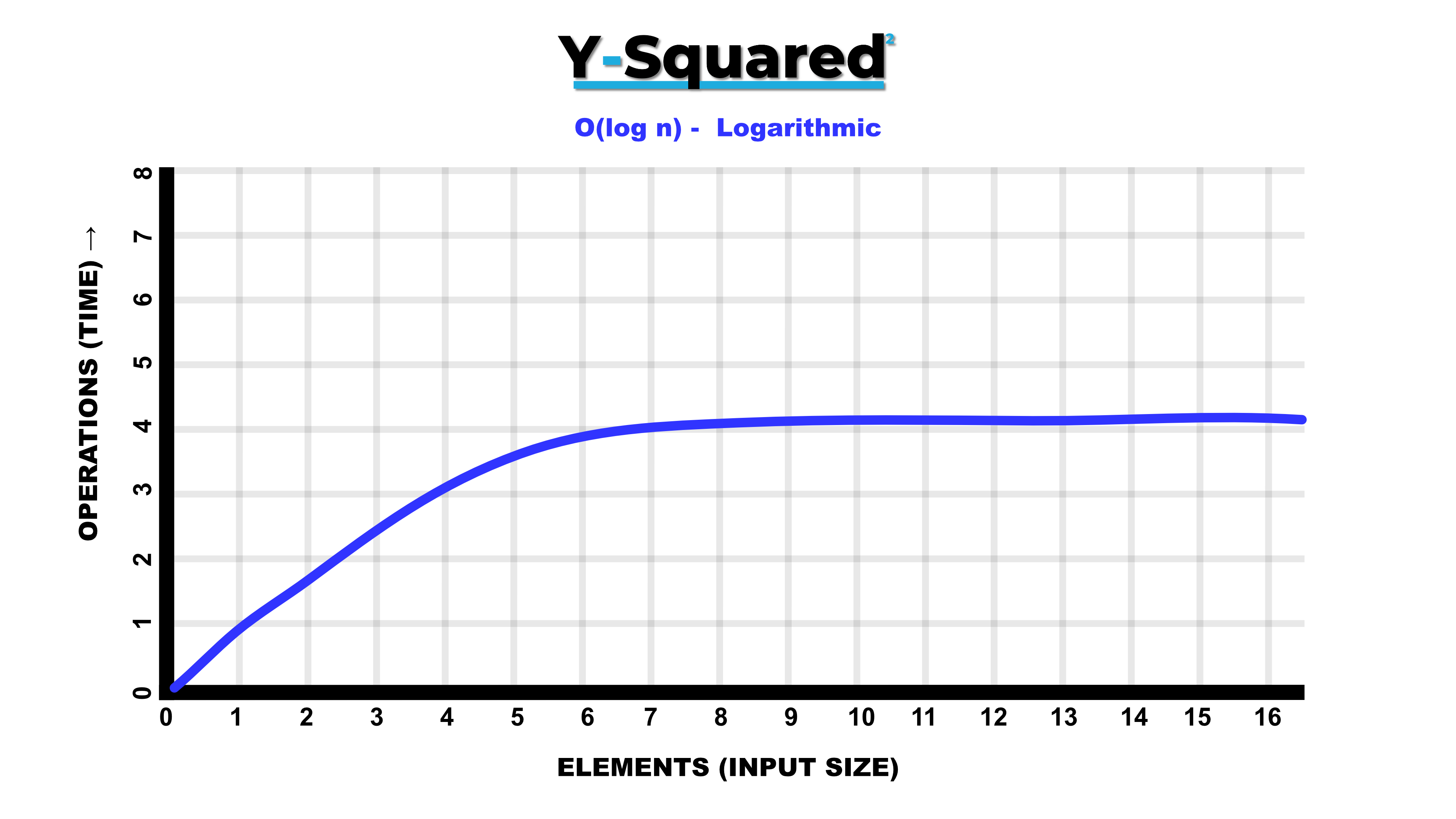We usually find O(log n) in algorithms that use divide and conquer techniques such as binary search or quicksort if they are sorted already. An example of a sorted list would be an array of names in alphabetical order.

How do divide and conquer techniques work? For example when using a binary search we repeatedly divide the search of a sorted list into smaller and smaller intervals. Even though the input size is increasing each time, the time complexity actually gets more efficient.

Binary search code example of O(log n):

``````let binarySearchExample = function (array, value) {
let start = 0;
let end = array.length - 1;
while (start <= end) {
let middle = Math.floor((start + end) / 2);
if (array[middle] === value) return true;
else if (array[middle] < value) start = middle + 1;
else end = middle - 1;
}
return false;
};

let ourArray = Array.from({ length: 1000 }, (_, i) => i + 1);

if (binarySearchExample(ourArray, 150, 0, ourArray.length - 1))
console.log("Element found!");

if (binarySearchExample(ourArray, 10000, 0, ourArray.length - 1))
console.log("Element found!");
``````

So how do we define O(log n) once and for all? I read a response to a question on Stack Overflow not too long ago that has stuck with me.

“O(log n) basically means time goes up linearly while the n goes up exponentially. So if it takes 1 second to compute 10 elements, it will take 2 seconds to compute 100 elements, 3 seconds to compute 1000 elements, and so on. It is O(log n) when we do divide and conquer type of algorithms e.g binary search. Another example is quick sort where each time we divide the array into two parts and each time it takes O(N) time to find a pivot element. Hence it N O(log N)”

User - FastcodejavaLink to original post****

## 8 O(n log n) - Log Linear

So what is 0(n log n), it is literally n linear time, multiplied by log n logarithmic time. Usually this can be found in sorting algorithms

What about dropping non-dominant terms in Big O? This is generally when we are adding two different time complexities, but in 0(n log (n)) we are using multiplication. We can’t simplify n * log n any further so we keep it the way it is !

O(n log n) is not great in terms of efficiency but performs better than O(n^2) - Quadratic time but not as well as O(n) - Linear time.

Code example:

``````function logLinear(value) {
for (let i = 0; i < value; i++) {
// This loop is executed n times, so O(n)
for (let j = 1; j < value; j = j * 2) {
// This loop is executed O(log n) times
console.log("Running test!");
}
}
}
``````

## 9 O(2^n) - Exponential Time Complexity

Usually seen in recursive algorithms that solves a problem of size n.

An algorithm with exponential time complexity doubles in size with each addition to the input The time complexity begins with a lower level of difficulty and gradually increases till the conclusion.

O(2^n) is terrible in terms of time complexity.

Example code:

``````function fibonacciSequence(number) {
if (number <= 1) return number;
return fibonacciSequence(number - 1) + fibonacciSequence(number - 2);
}
``````

## 10 O(n!) - Factorial Time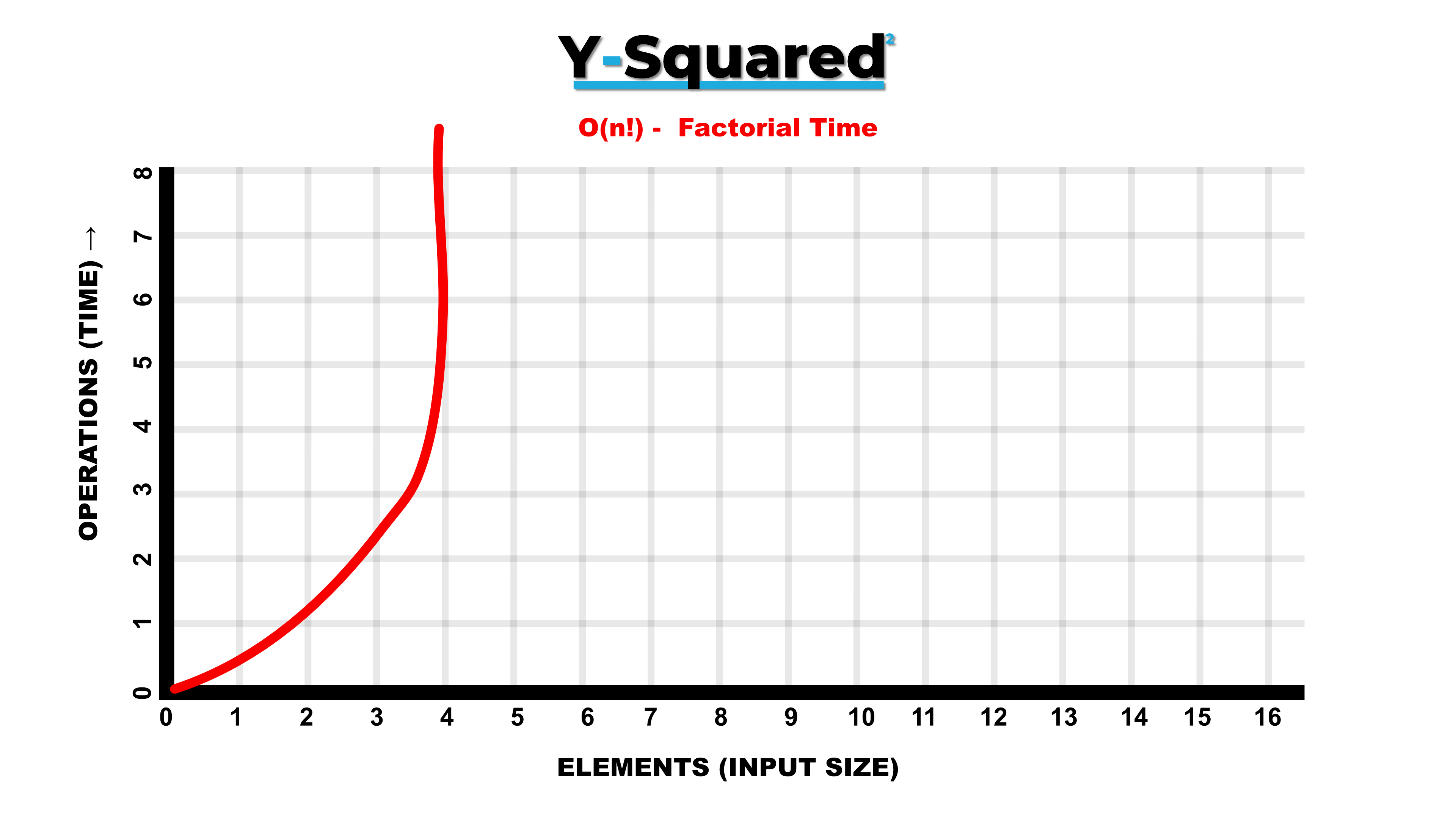O(n!) or factorial time is the worst rated for efficiency of all the Big O’s.

Operations on the y axis grow extremely fast and become extremely slow and unusable for even small values of inputs on the x axis.

Factorial time complexity is the multiplication of all numbers from n less than or equal to the given number other than (0).

3! - 1*2*3 = 6

6! - 1*2*3*4*5*6 = 720

20! = 2432902008176640000

Example code:

``````function factorialTime(number) {
if (number === 0) {
console.count(number);
return;
}
for (let i = 0; i < number; i++) {
factorialTime(number - 1);
}
}
factorialTime(3); //6
// factorialTime(6); //720
// factorialTime(8); //40320
``````

As you can see this gets out of hand pretty quickly.

## 11 Space Complexity

So far we've been talking about time complexity, the other side of Big O is memory or space complexity. This part is a lot easier to wrap your head around but it can catch people out as the time complexity can be different to the space complexity, it uses the same notation but the results may vary.

• Speed - Big O Time Complexity - Time efficiency
• Memory - Big O Space Complexity - Memory efficiency

Something to keep in mind going forward is that there is usually a trade off between space and time.

In real life we are inevitably limited by memory, so what causes space complexity ?

• Variables
• Data Structures
• Function Call
• Allocations

Let’s look at some examples:

Example 1:

Time complexity and space complexity are different in the below example the space complexity is 0(1) even though in time Complexity its O(n).

The reason this is O(1) - space complexity constant, is because within the function we are not adding anymore space.

``````function toto(n) {
for (let i = 0; i < n; i++) {
console.log("toto");
}
}
// O(1) Space Complexity - Constant
``````

Example 2:

Space complexity is O(n) in this example because we are creating a new variable and adding a new data structure, so we are creating more space, O(n) - Space Complexity Linear.

``````function arrayOfTimes(n) {
let hiArray = [ ]; // New Variable
for (let i = 0; i < n; i++) {
// Loop
hiArray[i] = "hi"; // New Data Structure
}
return hiArray;
}
// O(n) Space Complexity - Linear
// Note: Like time complexity we drop the constants so O(1 + n) becomes O(n)
``````

## 12 What’s next?

This is the first of many blog posts I hope to write on https://y-squared.blog/ and Medium. Hopefully you’ve found this post valuable!

In part two we will take a deep dive into data structures.

## 13 Contact, Links & GitHub Repo

Please fill out the form on contact form or email us at team@y-squared.com.

The Author:This post was written by Robert from Y-Squared: